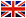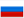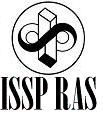Methods of the Fermi-liquid description

E.V. Deviatov, PhD

1. Introduction. Electron-electron interaction
2. Landau theory of the Fermi-liquid. Kinetic equation.
3. Some aspects of quantum mechanics. Secondary quantization, Fermi golden rule.
4. Hartree-Fock approximation I. BCS theory of superconductivity.
5. Formalism of the response functions. Dynamic form-factor, density-density response function.
6. Fermi-liquid of charged particles. Screening. Dielectric function.
7. Hartree-Fock approximation II. Random phase approximation. Habbard corrections.
8. Kubo-greenwood formula. One-dimensional transport, Landauer formalism. One-parameter scaling hypotheses.
9. Dielectric susceptibility of a two-dimensional electron gas in RPA approximation. Friedel oscillations. Scattering of electrons by charged impurities.Agarkov D.A. • Tel: +7(916)7584930 • email: agarkov@issp.ac.ru